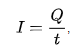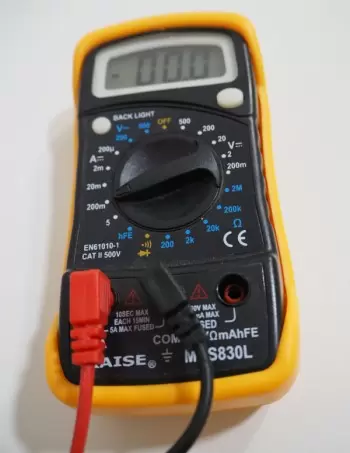# What Is Electric Current?

Electric current is the transport of electric charge. This transport is carried out mainly through the movement of electrons through conductors and semiconductors under the influence of a potential difference.In the field of electricity, the movement of ions in an electrolyte or plasma also causes an electric current. In all these cases, the transportation of cargo is carried out by the movement of electrical charges. In addition, an electric current is also created as a change in electric flux, such as between the plates of a capacitor during charging and discharging, without moving the charge carriers.

## Direction and Strength of Electric Current

In dilute gases, electrolytic solutions, and molten electrolytes, the positive and negative ions move in opposite directions. In a metal conductor, negatively charged free electrons move from the negative pole (surplus of electrons, and therefore of lower potential) to the positive pole (electron deficiency, with a higher potential).

Traditionally, electric current is expressed as the displacement of the positive charge. When it was learned that electric current is generally caused by electrons moving in the opposite direction, the electron was by definition assigned a negative charge. The old definition of flow direction, therefore, remained in force.

## Amperage

Electric current is usually shown with the letter I (for current intensity) and can be described as the displacement of the electric charge per unit time, that is, in coulomb (C) per second (s).

For a constant force current:where:

• I is the electric current is expressed in amps (A).
• t the considered time, expressed in seconds (s).
• Q the time t amount of electric charge moved expressed in coulomb (C).

The intensity of the electric current is measured in amps (symbol: A), and informally it is also known as amperage by analogy with the voltage of the electrical voltage.

## How Is the Current Measured?

The most used devices to measure an electric current are:

• Amperímetro.
• Clamp meter.An ammeter is used to measure the electric current. The ammeter is connected in series with the circuit to be measured. In order not to influence too much the circuit to be measured, the instrument should cause the least possible loss of voltage.

Another method of measuring electric current is with a current clamp or a current clamp.

An ammeter clamp is a measuring instrument for alternating current, designed as a clamp that is clamped around a current carrying conductor. In clamps, the magnetic field created around the conductive material induces a current, which is a measure of the current flowing through the attached conductor.

## What Are the Types of Electrical Currents?

The different types of current are as follows:

1. DC direct current.
2. AC alternating current.
3. Sinusoidal current.
4. Quasi-stationary current.
5. High frequency electric current.
6. Eddy current.
7. Single-phase current.
8. Triphasic current.

### DC

Direct current is a current whose direction and magnitude do not change over time.

The electricity provided by the solar panels in a photovoltaic solar energy installation is obtained in direct current.

### Alternating Current

Alternating current is an electrical current that varies over time.

Any current that is not constant would fall within this classification. Power inverters in a photovoltaic solar installation are in charge of converting the electricity obtained thanks to the photovoltaic effect into alternating current.

### Sinusoidal Current

Sinusoidal current is a periodic electric current, which is a sinusoidal function of time. Among the alternating currents, the main one is the current, whose value varies according to a sinusoidal law. In this case, the potential of each end of the conductor changes with respect to the potential of the other end of the conductor alternately from the  positive pole to the negative pole and changes direction, passing through all the intermediate potentials (including the zero potential).

The result is a current that changes direction continuously: when it moves in one direction, it increases, reaching a maximum, called the amplitude value, then it decreases, at some point it becomes equal to zero, then it increases again, but in the other direction. and it also reaches its maximum value. Thereafter it decreases to zero again, after which the cycle of all changes resumes.

### Quasi-stationary Current

Quasi-stationary current is "a relatively slow-changing alternating current, for whose instantaneous values ​​the laws of constant currents are followed with sufficient precision. These laws are Ohm's law, Kirchhoff's rules, and others. Quasi-stationary current, such as current Continuous, it has the same intensity of current in all sections of an unbranched circuit.

Induction capacitance and inductance are taken into account as grouped parameters.

Normal industrial currents are almost stationary, with the exception of currents in long distance transmission lines where the quasi-stationarity condition along the line is not met.

Electromagnetic disturbances propagate along the electrical circuit with the speed of light, therefore, for currents that change periodically. For example, a 50 Hz power frequency current is almost stationary for circuits up to 100 km in length.

### High Frequency Electric Current

A high-frequency electric current is an alternating current (starting at a frequency of about tens of kHz), for which phenomena, such as electromagnetic radiation and the skin effect, become significant.

If the wavelength of alternating current radiation becomes comparable to the dimensions of the elements of the electrical circuit, the quasi-stationary condition is violated, which requires special approaches to the calculation and design of such circuits.

### Eddy Current

Eddy currents are closed electrical currents in a massive conductor that occur when the magnetic flux that penetrates it changes, making eddy currents induction currents. The faster the magnetic flux changes, the stronger the eddy currents will be.

The existence of eddy currents leads to an effect on the skin, that is, to the fact that alternating electric current and magnetic flux propagate mainly in the surface layer of the conductive material. Eddy current from conductors leads to energy losses, especially in the cores of alternating current coils.

To reduce energy losses by eddy currents, division of alternating current magnetic circuits into separate plates isolated from each other and located perpendicular to the direction of eddy currents is used. This separation limits the possible contours of your paths and greatly reduces the magnitude of these electrical currents.

At very high frequencies, instead of ferromagnets, magneto-insulators are used for magnetic circuits, in which, due to the very high resistance, the eddy currents practically do not occur.

### Single Phase Current

A single-phase current is made up of a single alternating current or phase and therefore all the voltage varies in the same way.

Single-phase electricity distribution is often used when the loads are mainly for lighting and heating, and for small electric motors.

### Triphasic Current

A three-phase current is a set of three single-phase alternating currents of equal frequency and amplitude, which have a phase difference of 120 ° between them, and are given in a certain order.

Each of the single-phase currents that make up the system is designated with the name of phase.

The three-phase system presents a series of advantages, such as

1. The economy of its energy transmission lines and the transformers used.
2. The high performance of the receivers, especially motors, to which the three-phase line feeds with constant power.

The generators used in power plants are three-phase, since the connection to the electrical network must be three-phase (except for low-power plants). The three-phase is used massively in industries, where the machines work with three-phase motors.

### Other Types of Electric Current

There are other types of current that are also worth mentioning:

• The ripple current is a periodic electric current, the average value of which over a period is different from zero.
• Unidirectional current is an electric current that does not change its direction.
• The periodic current is an electric current, the instantaneous values ​​of which are repeated at regular intervals in an unchanged sequence.

At the same time, other classifications can be made:

If the charged particles move within the macroscopic bodies relative to a particular medium, that current is called the conducting electric current. If the particles move macroscopic charged bodies (for example, charged raindrops), this current is called convection.

Author:

Published: October 7, 2019
Last review: October 7, 2019